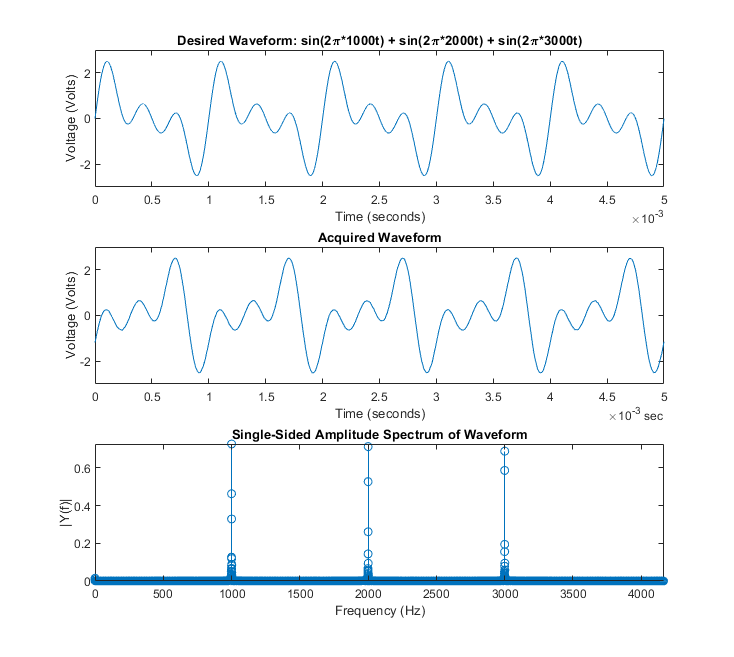# Generate Arbitrary Periodic Waveforms Using Digilent Analog Discovery

Use function generator channels to generate an arbitrary 1kHz waveform function, and record data at the same time, using an analog input channel.

### Discover Digilent Devices

Discover Digilent devices connected to your system using daqlist.

daqlist("digilent") dq = daq("digilent") 
ans = 1×4 table DeviceID Description Model DeviceInfo ________ _____________________________________________ ____________________ _______________________ "AD1" "Digilent Inc. Analog Discovery 2 Kit Rev. C" "Analog Discovery 2" [1×1 daq.di.DeviceInfo] dq = DataAcquisition using Digilent Inc. hardware: Running: 0 Rate: 10000 NumScansAvailable: 0 NumScansAcquired: 0 NumScansQueued: 0 NumScansOutputByHardware: 0 RateLimit: [] Show channels Show properties and methods 

### Add a Function Generator Channel

Add a function generator channel with device ID AD1 and channel ID 1. Set the waveform type to Arbitrary. The voltage range of the output signal is -5.0 to +5.0 volts.

ch_fgen = addoutput(dq, "AD1", "1", "Arbitrary"); ch_fgen.Name = "AD1_1_fgen" 
ch_fgen = Index Type Device Channel Measurement Type Range Name _____ ______ ______ _______ ________________ ____________________ ____________ 1 "fgen" "AD1" "1" "Arbitrary" "-5.0 to +5.0 Volts" "AD1_1_fgen" 

### Define a Sum of Sinusoids as the Output Waveform

The function generator produces periodic outputs by repeatedly generating the contents of its buffer (4096 points). A waveform is constructed to fill this buffer without repetition.

buffersize = 4096; len = buffersize + 1; f0 = 1; f1 = 1 * f0; f2 = 2 * f0; f3 = 3 * f0; waveform = sin(linspace(0, 2*pi*f1, len)) + ... sin(linspace(0, 2*pi*f2, len)) + ... sin(linspace(0, 2*pi*f3, len)); waveform(end) = []; 

### Assign the Waveform Data and Set Waveform Frequency

frequency = 1000; ch_fgen.WaveformData = waveform; ch_fgen.Frequency = frequency; 

Add an analog input channel with device ID AD1 and channel ID 1. Set the measurement type to Voltage.

ch_in = addinput(dq, "AD1", "1", "Voltage"); ch_in.Name = "AD1_1_in" 
ch_in = Index Type Device Channel Measurement Type Range Name _____ ____ ______ _______ ________________ __________________ __________ 1 "ai" "AD1" "1" "Voltage (Diff)" "-25 to +25 Volts" "AD1_1_in" 

### Define the Acquisition Scan Rate

Acquire data at a higher scan rate than the highest frequency in the generated waveform (oversampling).

oversamplingratio = 50; Fn = 2 * frequency; Fs = oversamplingratio * Fn; dq.Rate = Fs; 

### Generate a Periodic Waveform and Record Input

data = read(dq, seconds(3)); 

### Define Plot Parameters

k = 5; width = 750; height = 750; period = 1/frequency; numperiods = k * period; maxamplitude = 3*ch_fgen.Gain; wavedesired = repmat(waveform', k, 1); tsamples = linspace(0, numperiods, k * buffersize)'; 

### Define FFT Parameters

L = 2 * oversamplingratio * buffersize; NFFT = 2^nextpow2(L); Y = fft(data.AD1_1_in, NFFT)/L; f0 = (Fs/2) * linspace(0, 1, NFFT/2 + 1); 

### Plot Waveforms

plotScaleFactor = 12; plotRange = NFFT/2; % Plot is symmetric about NFFT plotRange = floor(plotRange / plotScaleFactor); Yplot = Y(1:plotRange); fplot = f0(1:plotRange); fig = figure; % Plot Desired Waveform subplot(311) plot(tsamples, wavedesired); xlabel('Time (seconds)'); ylabel('Voltage (Volts)'); title('Desired Waveform: sin(2\pi*1000t) + sin(2\pi*2000t) + sin(2\pi*3000t)'); xlim([0 numperiods]); ylim([-maxamplitude maxamplitude]); % Plot Acquired Waveform subplot(312) plot(data.Time, data.AD1_1_in); xlabel('Time (seconds)'); ylabel('Voltage (Volts)'); title('Acquired Waveform'); xlim([seconds(0) seconds(numperiods)]); ylim([-maxamplitude maxamplitude]); % Plot Single-Sided Amplitude Spectrum subplot(313) stem(fplot, 2*abs(Yplot)); title('Single-Sided Amplitude Spectrum of Waveform') xlabel('Frequency (Hz)') ylabel('|Y(f)|') axis tight % Make Graph Larger outpos = get(fig, 'OuterPosition'); set(fig, 'OuterPosition', [outpos(1)-125 outpos(2)-375 width height]);